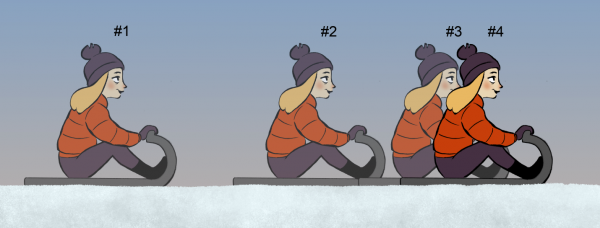# Physics in Animation: Force and Momentum

Continuing in the series on Physics in Animation, today we take a closer look at Force and Momentum. How can these principals help you to take your work to the next level?

As discussed in Parts 1 and 2, the Principles of Animation Physics are helpful for animators looking to create physically believable motion. These principles are:

#### Principle #3: Force and Momentum

To understand how forces, such as gravity, friction, etc., affect motion you first need to understand the concept of momentum. Moving objects have momentum, which depends on both their speed and weight. A character that weighs 100 pounds (45 kilos) can have as much momentum as a 300 pound character if the small character runs three times faster than the big guy. Momentum also depends on the direction of the motion so deflecting a moving object is considered a change of momentum.

The Law of Inertia (Principle #2) tells us that we have uniform motion (constant timing and spacing with a straight path of action) when there’s no unbalanced forces. The principle of Force and Momentum says that an unbalanced force will change an object’s momentum; the bigger the force, the quicker the momentum changes.  For moving characters this change in momentum could result in increasing or decreasing a character’s speed or it could be a change in direction (such as making a turn). Among physicists the principle of Force and Momentum is known as Newton’s Second Law of Motion.

It’s also called the Law of Acceleration; you may know it by its equation:  F = m a.So, as an animator, how do you use this principle? Let’s say that you want to indicate that a large force is acting on a character, such as a strong punch in a fight scene. The punch (which is our unbalanced force) should cause a quick change in momentum. For example, you can have the character accelerating quickly as he recoils from the punch. Or if the character was already moving when the punch landed then the force could quickly stop his motion. In both cases we have a change in momentum and the larger the force, the quicker this change occurs. Remember that momentum depends on both velocity and weight so a heavy character recoils less than a light character hit by the same punch. This is a great way to convey a sense of weight for characters by showing how they react to forces.Here’s another example: a character is sliding to a stop due to friction, which is the unbalanced force. When this friction is small the momentum changes slowly which means it takes a long time for the character to come to a stop.  The principle of Force and Momentum also tells us that if the force is constant (which is typical for sliding friction) then the change in momentum produces spacings that follow the Odd Rule (see the principle of Timing, Spacing, and Scale in Part #1).Often the unbalanced force is not constant. If the unbalanced force is increasing then the spacings change faster than the Odd Rule. On the other hand, if the unbalanced force is decreasing then the spacings become more uniform. For example, when Tarzan is swinging from tree to tree his speed is nearly constant near the bottom of the arc. In that part of the swing the downward force of gravity is almost balanced by the upward tension in the vine. Tarzan’s momentum is large, but it changes very little during that lowest part of the swing. Shoot some reference and see for yourself!

Besides timing and spacing, forces also affect the path of action; as mentioned above a change in direction is considered a change in momentum. So if you have a pair of characters running towards each other and colliding (such as in a football match), the greater the deflection of their motion, the greater the force of impact. And remember that a character’s momentum depends on weight so a light character experiences a greater deflection from this impact than the heavy character. We’ll discuss this further in Part 6 (Action / Reaction).

Finally, be sure to use the principle of Force and Momentum when you’re studying reference. While watching motion, try to think in terms of how momentum is changing and the forces causing this change. Some forces, such as a punch to the head, are easy to see while others, such as sliding friction, aren’t as obvious. But all forces have an effect on motion and all motion that’s not uniform has unbalanced forces.  Both the timing and the path of action depend on these unbalanced forces so your animated motion will be believable only if the texture of the timing and the shape of the path of action are consistent with the forces at play. In brief: Use the force! (You knew that was coming. )

That’s all for now. Look for future articles covering the other Principles of Animation Physics appearing here on Animator Island. See you then!## Similar Posts

Subscribe
Notify of